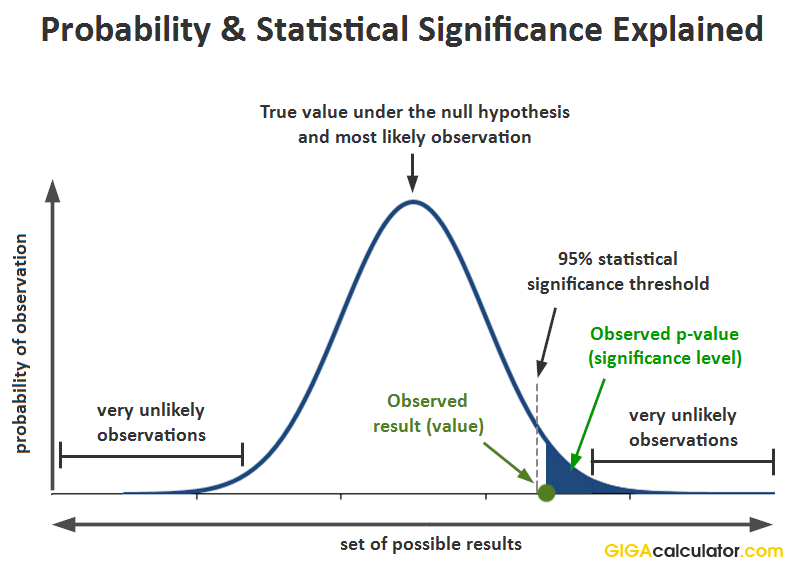# Chi-Square to P-value Calculator

Use this Χ2 to P calculator to easily convert Chi scores to P-values and see if a result is statistically significant. Information on what a p-value is, how to interpret it, and the difference between one-sided and two-sided tests of significance.

Χ2 =
Share calculator:

Embed this tool:
get code

## Using the Chi Square to p-value calculator

Having obtained a Χ2 statistic from a given set of data you would often want to convert it to its corresponding p-value. This Chi Square to P-value calculator is easy to use and requires minimum input to get the job done. Simply enter the Chi-Square statistic you obtained and the degrees of freedom: N-1 for one-dimensional calculations, (Ncols - 1) * (Nrows - 1) for multiple columns/groups, then choose the type of significance test to calculate the corresponding p-value using the Χ2 CPDF (cumulative probability density function of the chi-square distribution).

## What is a "p-value"

The p-value is used in the context of a Null-Hypothesis statistical test (NHST) and it is the probability of observing the result which was observed, or a more extreme one, assuming the null hypothesis is true 1. In notation this is expressed as:

p(x0) = Pr(d(X) > d(x0); H0)

where x0 is the observed data (x1,x2...xn), d is a special function (statistic, e.g. calculating a Chi-Square score), X is a random sample (X1,X2...Xn) from the sampling distribution of the null hypothesis. This can be visualized in this way:In terms of possible inferential errors, the p-value expresses the probability of committing a type I error: rejecting the null hypothesis if it is in fact true. The p-value is a worst-case bound on that probability. The p-value can be thought of as a percentile expression of a standard deviation measure, which the Z-score is, e.g. a Z-score of 1.65 denotes that the result is 1.65 standard deviations away from the arithmetic mean under the null hypothesis. Therefore, one can think of the p-value as a more user-friendly expression of how many standard deviations away from the normal a given observation is. Since the Chi-Square distribution is one-tailed and varies with the degrees of freedom you specify (higher degrees of freedom resulting in significantly fatter right tail) the p-value can always be visualized as cutting a slice from the right tail of the distribution.

## How to interpret a low p-value from a Chi Square test

Saying that a result is statistically significant means that the p-value is below the evidential threshold decided for the test before it was conducted. For example, if observing something which would only happen 1 out of 20 times if the null hypothesis is true is considered sufficient evidence to reject the null hypothesis, the threshold will be 0.05. In such a case, observing a p-value of 0.025 would mean that the result is statistically significant.

The Pearsons's Chi-Square test can be used as a goodness-of-fit test for IID data (Independent and Identically-Distributed) and can thus be an omnibus test for independence and homogeneity. As a goodness-of-fit test it is used to compare the distribution of a set of data to a distribution which the data is stipulated to be generated from. One can easily see how by replacing the hypothetical distribution with an actual empirical one the test in its two-sample form turns to a classic NHST for determining if two samples come from the same distribution.

Let us examine what inferences are warranted when seeing a result which was quite improbable if the null was true. Observing a low p-value can be due to one of three reasons :

1. There is a true effect from the tested treatment or intervention. When used as a goodness-of-fit test: the empirical distribution does not fit the theoretical one due to it resulting from a data generating process resulting in non-normal, dependent or heterogeneous data.
2. There is no true effect, but we happened to observe a rare outcome. The lower the p-value, the rarer (less likely, less probable) the outcome. As a goodness-of-fit: the empirical distribution fits the theoretical one, but we reject the fit in error.
3. The statistical model is mis-specified (does not reflect the actual data generating mechanism). This can happen if the Chi-Square distribution does not describe the parameter of interest.

Obviously, one can't simply jump to conclusion 1.) and claim it with one hundred percent certainty, as this would go against the whole idea of the p-value. In order to use the p-value as a part of a decision process you need to consider external factors, which are a part of the experimental design process, which includes deciding on the significance threshold, sample size and power (power analysis), and the expected effect size, among other things.

If you are happy going forward with this much (or this little) uncertainty as is indicated by the p-value, then you have quantifiable guarantees related to the effect and future performance of whatever you are testing. When used as a goodness-of-fit test it should be noted that a high p-value does not necessarily mean the assumed theoretical distribution can be used since the chi-square test might result in a low p-value due to low sensitivity (power).

## Chi Square to P-value conversion table

Below are some commonly encountered standard scores and their corresponding p-values, assuming a two-sided hypothesis and 1 degrees of freedom.

Standard score to p-value table
Chi Square (Χ2)P-value
1.6424 80%
2.7057 90%
3.8416 95%
4.2177 97.5%
5.4117 98%
9.5493 99%
10.8274 99.9%
12.115 99.95%

Below is a table with chi square values and their corresponding p-values, assuming a two-sided hypothesis and 2 degrees of freedom.

Standard score to p-value table
Chi Square (Χ2)P-value
3.2189 80%
4.6052 90%
5.9915 95%
6.4378 97.5%
7.824 98%
12.43 99%
13.817 99.9%
15.199 99.95%